## General Chemistry

Learn the toughest concepts covered in Chemistry with step-by-step video tutorials and practice problems by world-class tutors

21. Nuclear Chemistry

Radioactive reactions deal with the chemical instability of the nucleus in an atom.

Nuclear Reactions

Heavy (large atomic mass) elements undergo radioactive reactions in order to increase the stability of their nuclei.

1
concept

## Types of Radiation Concept 12m
Play a video:
Hey, guys, In this new video, we're gonna take a look at nuclear reactions. Now we're going to say here that nuclear reactions deal with chemical processes that take place in unstable nuclei atoms. Now, remember your basic picture of the atom we have spinning around the nucleus are electrons within our nucleus. We have our protons and neutrons are protons air positively charged are neutrons are neutral now, nuclear reactions deals with us somehow affecting the number of protons within our given. Adam, Now we're gonna say this normally happens with very large, bulky radioactive types of elements. Now, we're gonna stay here. Unlike normal chemical reactions where the identities of the elements stay the same, we're gonna say nuclear reactions often result in elements changing into completely different elements. So we're all used to stalk geometry and balanced chemical equations. For example, we're used to seeing we have H two gas here, plus n two gas here combined to give us NH three gas over here and balancing it. Here we put there's a two here we put it to here and a three here, but for nuclear reactions were actually affecting the number of protons within our element. Remember, your protons or your atomic number represents the identity of that element. Every element has its own unique atomic number that no other element has. But a nuclear reactions were actually messing around with the number of protons, which results in us creating completely new and different elements. So you could start out with calcium 20 and somehow go through some process in which calcium 20 I'm calcium 40. I mean becomes are gone. So that's the whole basis of nuclear reactions. We go from one element to a completely new element. By affecting the number of protons affecting number of protons has a direct impact on the identity of the element.

In a typical stoichiometric reaction we begin with elements as reactants and end with the same elements in different forms as products.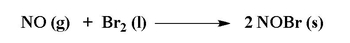In a nuclear reaction the number of protons in an element are affected and so the identity of the element changes.The three most common types of radioactive reactions are alpha decay or capture, beta decay or capture and gamma emission

2
concept

## Types of Radiation Concept 23m
Play a video:
Now we're gonna say here, when it comes to nuclear reactions, we can think the British physicist Ernest Rutherford, who really did a lot of experiments with nuclear reactions His contribution to nuclear chemistry was so great that they actually named Element 104 after him. Element 104 is called Ruth or 40. Um So it's kind of Ahm bridge toe all the work that he's done in terms of this field. Now, Rutherford basically broke down nuclear reactions into three major types of categories. We have our Alfa decay and you may hear instead of alpha decay, you may hear Alfa emission. We also have beta decay, which you may also here as beta emission. And then finally, we have gamma emission. You tend to just hear it is gamma mission. You usually don't hear the term gamma decay. Now what is the word decay or mitt me? Well, that means that the radioactive particle will be a product. So remember, if you hear the word decay or emit for a mission, that means that the radioactive particle involved in all these reactions will be a product. Now the opposite of decay or meeting would be the word capture, so capture will be the complete opposite. Capture would mean that the radioactive particle involved and each of these types of nuclear reactions would be a reacted. So in Alfa decay, we emit an alpha particle. In beta decay, we emit a beta particle and gamma emission. We emit a gamma particle. These particles are what caused our elements to go from one type to another type. And what you have to remember is when they say DK or emission. They're saying that this alpha particle beta particle or gamma particle will be a product. But if you hear the term capture or even absorption, that means that the alpha particle, the beta particles gamma particle, will be reacted. And this has a profound difference on what exactly your products will be because you'll be emitting or decaying these particles along with a whole new element with it. So the farther into into this chapter we go, we'll learn that beyond these three, we also have positron emissions as well as electron capture. Those who come after we learn these first three major types. So just remember, in a regular chemical reaction, we start out with Let's say carbon, we end with carbon. But in the nuclear reaction were emitting or capturing radioactive particles. And as a result, that's gonna change the identity of my element. You could start that with calcium and end up with something completely different, like our God.

In a radioactive decay or emission reaction the radioactive particle is ejected from the nucleus and forms a product.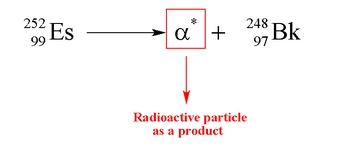In a radioactive capture or absorption reaction the radioactive particle is taken into the element and so is seen as a reactant.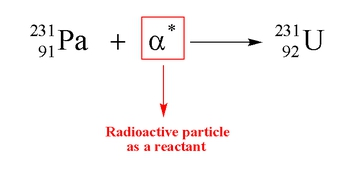Alpha Decay

An alpha particle is comprised of 2 protons and 2 neutrons.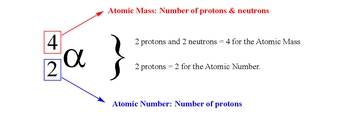3
concept

## Understanding Alpha Decay or Emission4m
Play a video:

An alpha decay or alpha emission occurs when an unstable nucleus ejects an alpha particle to create a new element.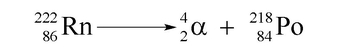4
concept

## Alpha Decay Concept 21m
Play a video:

The alpha particle is one of the largest radioactive particles with the highest ionizing power, but lowest penetrating power.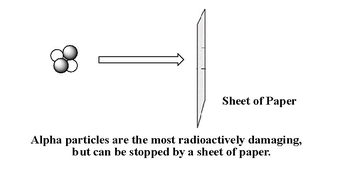5
example

## Alpha Decay Example 12m
Play a video:
Now here we have to write the balanced nuclear equations for each other following Alfa emissions. So for Alfie mission again, that's the same thing as a decay. That means that the alpha particle will be a product. So here we have Curium 2 48. Remember these numbers here are atomic masses. You have to actually look on your periodic table to find out the atomic number of curium and bismuth. So cure him on our periodic table is 96. We know that we're going to basically emit an Alfa particle, so it's gonna have to be a product again. We have four already, so we have to make sure that the new element next to it adds up to 2. 48 on the other side. So it's gonna be 2 44 plus plus. Forgive me to 48 and down here, this house to be 94. And what does that give me that gives me P U plutonium and then bismuth 207 That's the atomic mass. The weight on the bottom would be 83. So we have B I, we admit a helium or alfa particle. So we have four here but we need to 07 So we had two or three over 81 and that gives me t all. It's that simple. Now, if they were to ask an Alfa Absorption or Alfa Capture and that means that these alfa particles wouldn't be would not be any more products. They'd be reacting. So, for example, you have 40/20 which is calcium, and then you do Alfa capture, so I'd be on the same side. And then all you have to do is just add these numbers up so it would be 40 plus four is 44 over 22 to give you a new element. So you just look on your periodic table and see what element has an atomic number of 22 that would represent an Alfa capture.
Beta Decay

A beta particle has no atomic mass and is represented by an electron.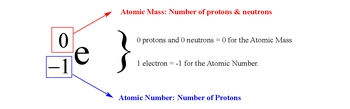6
concept

## Understanding Beta Decay or Emission1m
Play a video:
Hey, guys, In this new video, we're gonna take a look at beta decay. Now, we're gonna say that beta decay occurs when an unstable nucleus somehow emits a new electron. Now we're gonna say beta particle can be represented by E for the electron. I like trying here. We're gonna say much smaller than the other two subatomic particles. So the atomic mass can just be understood as zero. And here we're gonna say it. Atomic number is negative one because the atomic number is basically the number of protons. Since this is an electron, we're going to say that it's the opposite of a proton, which is one so an electron is negative one. Now, we're gonna say here beta decay can be represented when we emit a beta particle. So, for example, if we had Mercury and remember the 21 means that's its atomic math. So let's say we had mercury to one. If we look on our periodic table, Mercury has an atomic number off 80. We're gonna emit a beta particle. Now, remember, you're gonna say you're atomic masses have t equal each other on both sides of the arrow and your atomic numbers as well. Here. The electron has no mass. So the new element is gonna have a mass up to one. And then here we have to be very careful here. This is minus one. So minus one plus what gives me 80. Well, the answer would have to be 81 because 81 minus one gives me the 80 that I had originally. So just remember what? Remember that. So here would be t l. So this would be an example of a beta decay or a beta emission.

A beta decay or beta emission occurs when an unstable nucleus ejects a beta particle to create a new element.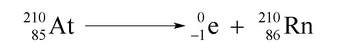7
concept

## Beta Decay Concept 242s
Play a video:
Now we're gonna say in terms of size, we know that the Alfa particles are the largest radioactive particle. So we're gonna say beta particles, therefore are smaller than alfa particles now, because they're smaller in size, they're gonna be less damaging if we ingest them. So here they're gonna have a lower ionizing power. But the problem here is because they're smaller, they're able to better penetrate into our skin. So here they're gonna have mawr penetrating power. And so to stop, you need a sheet of metal or large block of wood toe. Make sure beta particle does not enter inside of our bodies.

Beta particles are smaller in size, and therefore have more penetrating power. Luckily, they are less radioactively damaging because of their lower ionizing power.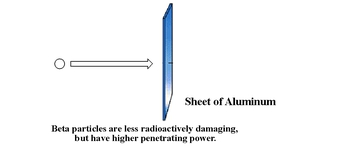8
example

## Beta Decay Example 11m
Play a video:
So here we have to write the balance Nuclear equations for each of the following beta emissions again. Beta decay. Beta emissions means that the beta particle will be a product. If they had said baited it capture or beta absorption, then it would be a reacted. So here we're starting out with magnesium 25. So that's the atomic mass. On our periodic table. Magnesium has an atomic number off 12. It's gonna emit a beta particle. So our new element that's being created would still have the same atomic mass. And then here, negative one. Plus what number gives me 12. It has to be 13 because 13 minus one gives me the 12 I started out with initially. So this element would be aluminum. Now, Ruffini, Um, are you one? 02 We're gonna see if we look in our periodic table roof in. IAM has an atomic number off 44. Again, we're gonna emit a beta particle, so this number stays 102 And this number here would have to be 45. So it goes upto Rh. Okay, so those were the examples off beta decays or beta emissions
9
example

## Beta Decay Example 26m
Play a video:
Gamma Emission

A gamma particle has no atomic mass and no atomic number and is represented by the sign gamma.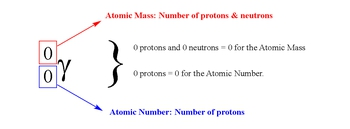10
concept

## Gamma Emission Concept 12m
Play a video:
Hey guys in this new video, we're gonna take a look at gamma emissions. So here we're gonna say gamma radiation is related to the electromagnetic spectrum. Now, we're gonna say here, gamma rays have the highest energy and therefore they have the lowest or shortest wavelength and then they have the highest frequency. Mhm. So remember you have to remember from electromagnetic spectrum theory that when it comes to energy, energy and frequency are directly proportional. All that means is if one is high, the other one would be high. But when it comes to wavelength, wavelength is inversely proportional to the both of them. All that means is that wavelength is the opposite of the other two. If they're high it's low If it's high they're low. So it's the complete opposite of frequency and energy. And remember wavelength is just the distance From one wave to another wave. Where frequency is how many waves do you get within 1/2? So if your distance between waves, the crest, the tops of them is very large. That means you don't get many waves in a second. But if the wavelengths are very small, if the distance between them is very small, You can cram a bunch of them in within 1/2. Okay, so then you would say the frequency is extremely high and the energy is high. Gamma rays have the highest behind cosmic rays, cosmic rays would actually be a little bit higher than gamma rays. We usually don't hear about this in lecture. But in lecture, So strictly lecture, we're gonna say gamma rays have the highest energy and therefore they have the highest frequency and therefore the lowest or shortest wavelength. If you went beyond just general, kem you go into physics and other higher level sciences. They start talking about cosmic rays, which are then even higher than gamma rays. But for right now, just focused on the simple electromagnetic spectrum, gamma rays are going to have the highest energy.

Gamma radiation is involved in the electromagnetic spectrum. Gamma rays possess the highest energy, while radio waves have lowest energy in terms of the spectrum.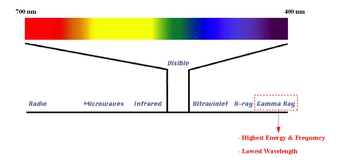11
concept

## Gamma Emission Concept 22m
Play a video:
now we're gonna say a gamma particle can be represented by 0/0. And the gamma symbol is this. Now you're gonna say because it's 0/0. You should realize that a gamma ray actually does not cause any change in your atomic mass or atomic number. And because of that we usually see it happening with alpha decay or beta decay. But what's the whole purpose of gamma mission then? Well we're gonna say when it comes to gamma mission it has to do with the absorption of energy. So here we're gonna say this wavy live line represents energy and this electron is in our first shell in our atom. So here in absorption the electron is gonna absorb that excess energy and become excited and use that extra energy just absorbed to jump up to either a higher shell number or to a higher orbital number. So basically if you go from one s to to us. So you're going from the first shell To the 2nd. Show that represents absorption. You could also go from the three s. 23 D. You can script skip three P. Altogether and just jump up straight to three D. Both of these examples represent absorption. The first one represents absorption when you jump from one shell to a higher shell and then the three S. The three D. Represents you absorbing energy and jumping up from a lower orbital to a higher orbital within the same shell. Both of them begin with the number three. So they're both within the third shell of your atom. But D orbital's have more energy than S orbital's. So if we saw this we'd have 40 over 20 calcium. It undergoes a gammy mission. And we'd say that that calcium has an electron that have just absorbed um energy and it's gonna become excited. So we put a little asterisks by it to show that it's in an excited state. So that would represent a gamma emission. Now we're gonna say that gamma particles they have the lowest ionizing power but they have the highest penetrating power. So if you're ever exposed to gamma mission like gamma radiation, it's basically a done deal, you're not gonna survive. Gamma radiation is extremely toxic to living tissue and biological systems. So any exposure to even the smallest amount of gamma radiation would completely eviscerate all the living cells and tissues within your body. So it has the lowest ionizing power, but it's still extremely dangerous.

The gamma particle does not create a new element like the other radioactive particles, but instead causes the excitation of electrons within an element.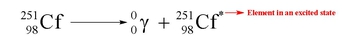Gamma Particles have lowest ionizing power, but are the most dangerous because of their highest penetrating power.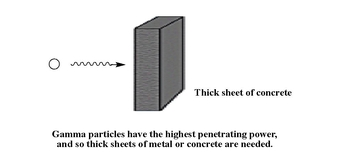12
example

## Gamma Emission Example 11m
Play a video:
Now if we go to this example, it says which of the following represents an element that has experienced a gamma emission. Here we have electron configurations for elements. Remember the normal pattern is you go from one S to to US 22 P. The three of us, the three P. For us etcetera. And remember gammy mission involves the excitation or the absorption of energy gets into an excited state. So you could actually have an electron skipping an entire orbital, Like it's supposed to be in the two s. But it gains so much energy, it skips to s. And goes to two p. Or you could have an electron gaining even more energy and going from two s. 23 s. So if we look the one that fits this excited state or this gamma emission state would have to be sodium because we're supposed to see one S followed by two S, followed by two P, Followed by three s. But what must have happened, this electron in here must have absorbed energy and therefore able to jump to a higher energy state. So the next one would be three p. So it skipped the three s. and went straight to three p. So this represents an element that has gained sufficient energy to become excited, which represents a gamma emission. So, remember Yemen mission may not change your atomic mass or atomic number like alpha decay or beta decay, but it still does affect the atom in some way
Electron Capture
13
concept

## Understanding Electron Capture1m
Play a video:
Hey, guys, In this new video, we're gonna take a look at electron capture. Now, the word capture we've been talking about this there is decay in a mission versus capture. Decane. A mission means that you're particle will be a product, but capture means that it'll be a reacted. And here we're talking about an electron. I remember an electron is really just a beta particle. So when we say electron capture, we're really saying beta capture. They're both dealing with an electron beta decay. The electron will be a product, but invade a capture or electron capture. It will be an reacted. So here we're gonna say electron capture involves the absorption, often electron, which remember, we saw as this symbol find unstable nucleus and is represented by the following reaction. So let's think of an example we could deal with Francie um, which is the metal most to the left and the lowest down group one A. So that's fr. And here we'll say we're dealing with isotope to 23. So here capture means that this electron is not gonna be a product, but it's gonna be a reacted. So we're gonna have Francie um 2 23. And Francine has an atomic number of 87. We're gonna absorb an electron. What effect is that gonna do? Well, it's gonna be 2. 23 plus zero. Gives me 2. 23 and then 87 minus one is gonna give me 86. So you become Radan. All right? Okay. So that represents the opposite of beta decay. Instead of doing beta decay, remission were doing beta capture, a k A. Electron capture.

In an electron capture or electron absorption reaction our electron particle is a reactant and not a product.

14
example

## Electron Capture Example 11m
Play a video:
now here. We have to say right balance nuclear equations for each of the following elements after undergoing electron capture. So remember Rutherford was the guy who came up with the three major types of nuclear reactions, and they named Element 104 after him. So here, Rutherford has an atomic number of 104 here. We're dealing with isotope to 63. We're gonna absorb an electron. So to 63 plus zero gives me to 63 104 minus one. Give me 103 And that will be L R for the next one. We're dealing with Nobel, Liam. Okay, so Nobel Prize, Nobel, Liam. So here, we're gonna have no belly, um, to 60 which is We absorbing electron. So to 60 plus zero is to 60 one or two minus one is one on one. That's M D. You'll find that a lot of elements around Nobel, you are named after states named after countries named after just inventors and physicists and scientists. So there's a lot of elements that are named after people we might have heard off or may not have heard off. So here we have 2 to 60/1. 01 m D. And finally we have led to 07 so this would be 207 The atomic number of lead is 82. We absorb an electron, so 207 plus zero is 207 80 to minus one is 81. So this would be an example of electron capture, which is the opposite of beta decay or a mission invaded a care admission. The electron is not a reactant but a product.
Positron Emission

A positron particle is referred to as the anti-electron particle because it looks like a positively charged electron.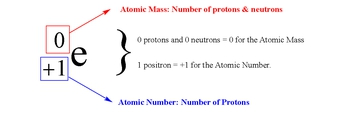15
concept

## Understanding Positron Emission1m
Play a video:
Hey, guys, In this new video, we're gonna take a look at positron emission. So here, we're gonna say positive positron emission occurs when an unstable nucleus emits a positron. Now, what's a positron? A positron is an anti particle of the electron. Remember, the electron is represented by this. Ah, positron is the opposite of that. So it looks like an electron. But instead of it having a negative sign, it'll have the opposite sign. So it'll be a positive electron. So a positron is considered just a positive electron. I know this is weird, but again, remember, we're dealing with nuclear reactions. So a lot of unaccustomed things that we are not used to seeing do occur. And one of them is this positron. So we're gonna say here, here's our positron now, because we're talking about the word emission again, Emission would mean decay, which means that this positron would be a product. So let's think of an example here. Einstein has its own element named after him Einstein E um, so Einstein E, um, we'll deal with isotope to 53 of Einstein E. Um, So Einstein, um, is yes. On our periodic table, it has an atomic number of 99 we're going to emit a positron. And because we're emitting a positron, let's see. So because the atomic masses zero the new elements still gonna be 53. But because the bottom is one what number plus one gives me 99 it have to be on 98. So here, that would just be CF. So this would be an example of a positron decay or positron emission.

A positron decay or positron emission occurs when an unstable nucleus ejects a positron particle to create a new element.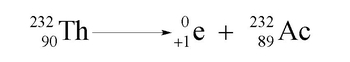16
example

## Positron Emission Example 11m
Play a video:
now, based on that, let's answer these two questions. It says right balance nuclear equations for each of the following positron emissions. So again, you're positron will be a product. So here we're dealing with uranium to 35 uranium is you on your periodic table. It's gonna have an atomic number of 92. We emit a positron. So this is gonna be 2 35 and 91 plus one. It gives me 92 so 91 would be P a. Now, next one is radon, which is RN. Okay. Radan has an atomic number of 86. We admit a positron. So now the elements still gonna be 2. 22 and 85 plus one gives me the 86. So that's act idiom. Okay, so these would be two examples off our positron emission
17
example

## Positron Emission Example 22m
Play a video: